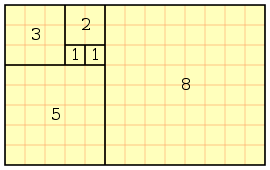# The Golden Ratio

Back in middle school art class, we learned about something called the “golden ratio.” It is basically a mathematical concept where two given numbers follow this basic rule: the ratio of the sum of the numbers to the larger of the two numbers is the same as the ratio of the larger number to the smaller number. The ratio is typically represented by the Greek letter phi (φ) and is equal to approximately 1.618.It gets really interesting when Fibonacci is involved. In the Fibonacci sequence, the first two numbers are 0 and 1, with each subsequent number being the sum of the previous two. For example, the first ten numbers in the sequence are 0, 1, 1, 2, 3, 5, 8, 13, 21, 34. Using this series of numbers, and creating arcs in each rectangle, one can create an approximate logarithmic spiral (though not a true logarithmic spiral, which involves a lot of mathematics that I don’t understand, though I really wish I did!).

So this:Which in turn can be found throughout the universe…

The following two tabs change content below.#### Kelli Ann Wilson

Kelli lives in rural New Hampshire with her husband Damian and their two children. She works as a writer, and in her free time enjoys reading, gardening, taking pictures, walking in the woods, and celebrating the seasons of nature and the feasts, festivals, and holy days of the Christian year.#### Latest posts by Kelli Ann Wilson (see all)